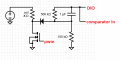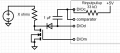# advice on a current measurment circuit

#### mikewax

Joined Apr 11, 2016
184
Here's a circuit i've designed for measuring approximate current flowing through a mosfet. I have a uC that has no ADC, it only has a comparator and an internal bandgap reference voltage. So i can't just connect the drain to an ADC input and divide the voltage by Rmosfet.The line marked "PWM" is not actually a PWM signal. It's just a regular high/low and starts off low, comparator disabled, DIO is high-Z. First,
1) DIO goes high & charges the cap for 500uS. So now Vcap = ~0.025v. Then
2) DIO goes high-Z, the comparator is enabled & PWM goes high long enough for the comparator to sense the voltage, then
3) comparator disables, PWM goes low
While the PWM was high, the drain voltage is
Vdrain = Icoil * Rds (0.033ohm). Suppose Icoil = 3A, then Vdrain = .099v
The comparator voltage then is
Vcomparator = Vdrain(~.099v) + .7 + Vcap (0.025v)
If Vcomparator(~0.824v) < Internal Bandgap Voltage (1.12v), then repeat at step 1 and increment Vcap by 0.025v
Eventually (after incrementing Vcap 13 times) Vcomparator > 1.12v, at which point i'll know that Vdrain = 1.12 - (.025 * 13) - .7 = 0.095v so
Icoil = 0.095 / 0.033 = ~2.88A which is close enough for my purposes.
My question is, is there any other way to rough measure Icoil with fewer components? I'm using 2 Rs, 1 cap & 1 diode. My circuit board is so damn tiny i'm having trouble fitting those 4 components.

#### Ian0

Joined Aug 7, 2020
8,972
Is there really no alternative version of the micro WITH an a/d?
Put the cap from DIO to ground, and a resistor in series with the diode to the MOSFET drain. Delete the other resistor.
Charge the cap up to V+ with the DIO output then make it high impedance. Measure the time taken for the voltage on the cap to reach the comparator threshold. The longer it takes, the higher the drain voltage, t varies with the logarithm of the voltage.
Don‘t forget that Rds(on) varies by at least a factor of 3 over temperature!

I’m assuming that Vdrain+Vdiode should not exceed Vref.
time taken will be
t=-R.C.ln((Vref-(Vin+Vdiode))/(Vcc-(Vdrain+Vdiode))

Last edited:
•mikewax

#### mikewax

Joined Apr 11, 2016
184
Is there really no alternative version of the micro WITH an a/d?
Put the cap from DIO to ground, and a resistor in series with the diode to the MOSFET drain. Delete the other resistor.
Charge the cap up to V+ with the DIO output then make it high impedance. Measure the time taken for the voltage on the cap to reach the comparator threshold. The longer it takes, the higher the drain voltage, t varies with the logarithm of the voltage.
Don‘t forget that Rds(on) varies by at least a factor of 3 over temperature!

I’m assuming that Vdrain+Vdiode should not exceed Vref.
time taken will be
t=-R.C.ln((Vref-(Vin+Vdiode))/(Vcc-(Vdrain+Vdiode))
thank you unfortunately there isn't. I searched the whole known universe & it's the only choice for now.
uuhhh.... you're saying to charge the cap all the way up and then discharge it through the diode-resistor-mosfet?
[5 minutes later]
ok YEAH i see what you mean & no Vdrain + Vdiode will never exceed Vref. That's a GREAT idea.
[5 minutes more]
OK the math works out. Much obliged[half hour]
hmmm.... if i charge the 1uF cap with a straight connection to the DIO, with a maximum rated current of 40mA, is that cool? The data sheet doesn't say anything about surge current of a DIO, they never do. But there IS a surge current capacity, just no way to know what it is. i better start a new thread for this....

Last edited:

#### mikewax

Joined Apr 11, 2016
184
OK i worked on it some more. Started plugging in the #s and the problem is that the voltage source on the left will burn out it has a very limited wattage. So i could not do the measure-the-cap-discharge method because it would take too long with as much as a ten-amp load on the V source. Also because i came up with another possible solution with fewer parts,
and now i have the following:initial: DIOc low, comparator disabled, DIOd low, DIOm low.
1) DIOd = Hi-Z, start counter
2) DIOc = input with pullup
3) DIOm = high
4) wait 4uS
5) DIOc = Hi-Z
6) enable comparator, if Vmosfet + Vdiode + Vcap > 1.1V reference, comparator will trigger
7) If it doesn't then counter++ and goto step 2. If it does, check counter and

using Ian0's formula (thanx),
counter * 4uS = -R.C.ln((Vref-(Vin+Vdiode))/(Vcc-(Vdrain+Vdiode)), and solve for Vdrain
and Idrain = Vdrain / Rds (36mohm, or check temp if i have to)
and X = 5 / Idrain